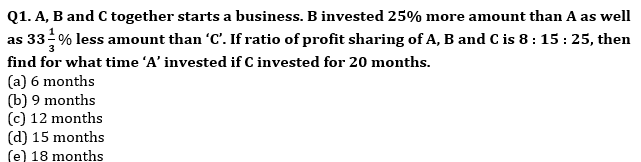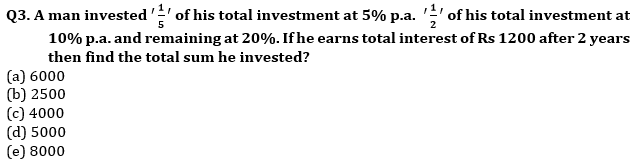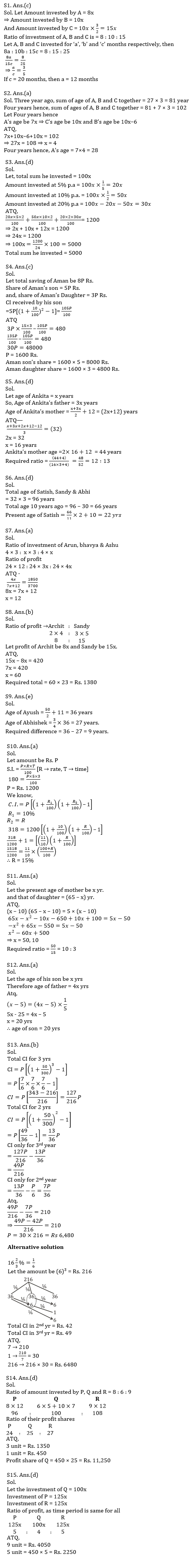Latest Banking jobs   »   Quantitative Aptitude Quiz For SBI PO,...

# Quantitative Aptitude Quiz For SBI PO, Clerk Prelims 2021- 18th JuneQ2. Three years ago, average age of ‘A’, ‘B’ and ‘C’ is 27 years. Four years hence, ratio of A and C’s age is 7 : 10. If B is 6 years younger than C, then find present age of ‘A’?
(a) 24 years
(b) 27 years
(c) 30 years
(d) 28 years
(e) 36 yearsQ4. Aman divided his total saving between his son and daughter in the ratio of 5 :3. His son invested his share on CI for two years at the rate of 10% and received the interest Rs. 480 less than his sister, who invested her share on SI for three years at the rate of 15% . Find Share of Aman’s son and daughter respectively?
(a) 10800 Rs, 2000 Rs
(b) 9600 Rs, 4200 Rs
(c) 8000 Rs, 4800Rs.
(d) 9800 Rs, 3000 Rs
(e) 7000 Rs , 5800 Rs

Q5. Ankita father’s age is two times more than her present age and age of Ankita’s mother is 12 years more than average of Ankita and her father’s age. If four years ago average age of all three was 32 years, then find the ratio between Ankita mother’s age and Ankita father’s age four years hence?
(a) 13 : 12
(b) 12 : 17
(c) 12 : 19
(d) 12 : 13
(e) 12 : 23

Q6. The average age of Satish, sandy & Abhi is 32 years and the ratio of their ages 10 years ago was 2 : 4 : 5 respectively . Find the present age of Satish?
(a) 16 yrs
(b) 12 yrs
(c) 10 yrs
(d) 22 yrs
(e) 18 yrs

Q7. Arun, Bhavya & Ashu entered into a partnership, ratio of investment of Arun & Bhavya is 4 : x and ratio of investment of Bhavya & Ashu is 3 : 4. If at the end of two years Ashu receives Rs 1850 as profit out of total profit Rs 3700. Then find the value of x?
(a) 12
(b) 14
(c) 16
(d) 8
(e) 10

Q8. Archit and Sandy enter into a business by investing in ratio 2 : 3 and the ratio of time period for which they invested is 4 : 5 respectively. If profit earned by Sandy is Rs. 420 more than the profit earned by Archit then find the total profit earned by Archit and Sandy both ?
(a) Rs. 1320
(b) Rs. 1380
(c) Rs. 1440
(d) Rs. 1280
(e) Rs. 1460

Q9. The age of Abhishek is ¾ th of the age of Ayush. The age of Ayush is 11 years more than the average age of his two sons whose total age is 50 years. Then find the difference of age of Abhishek and Ayush ?
(a) 14 years
(b) 6 years
(c) 12 years
(d) 8 years
(e) 9 years

Q10.An amount is invested at S.I. for three years at rate of 5% and S.I. received on that amount is Rs. 180. If the same amount is invested for two years at 10% C.I. for first year & R% C.I. for 2nd year & total C.I. received in two years is Rs. 318 then find value of R ?
(a) 15%
(b) 12%
(c) 22%
(d) 18%
(e) 25%

Q11. The sum of the ages of mother and daughter is 65 years. 10 years ago the product of their ages was 5 times the age of mother at that time. Then find the ratio of the present age of mother to the present age of daughter(age of mother is greater than 30 years).
(a) 10 : 3
(b) 12 : 5
(c) 9 : 2
(d) 3 : 1
(e) 4 : 1

Q12. The age of father is 4 times the age of his son. 5 years ago the age of son was 1/5 times of his father age. Find the present age of son.
(a) 20 yrs
(b) 30 yrs
(c) 28 yrs
(d) 25 yrs
(e) 32 yrs

Q13. Abhishek invested some amount for 3 yrs at rate of 16 ⅔% per annum at CI. The difference of CI obtained only on 3rd year and C.I. obtained only on second year is Rs 210. Find the amount invested by Abhishek?
(a) Rs 6,220
(b) Rs 6,480
(c) Rs 8,420
(d) Rs 7,420
(e) Rs 8,240

Q14. Three persons P, Q and R started a business by investing in the ratio of ⅔ :½ :¾ . After 5 months, Q increases his investment by ⅔rd of his initial amount. If after 12 months, the difference of the profit shares of P and R is Rs. 1,350 then find the profit share of Q.
(a) Rs. 11,500
(b) Rs. 11,200
(c) Rs. 12,250
(d) Rs. 11,250
(e) Rs. 10,250

Q15. P invested 25% more than Q and Q invested 20% less than R. If the time period of investment of all three is same and the sum of profit shares of P and Q is Rs. 4,050 then find the profit share of R.
(a) Rs. 2000
(b) Rs. 2150
(c) Rs. 2200
(d) Rs. 2250
(e) Rs. 2450

Practice More Questions of Quantitative Aptitude for Competitive Exams:

###### SBI Clerk Study Plan 2021

Solutions